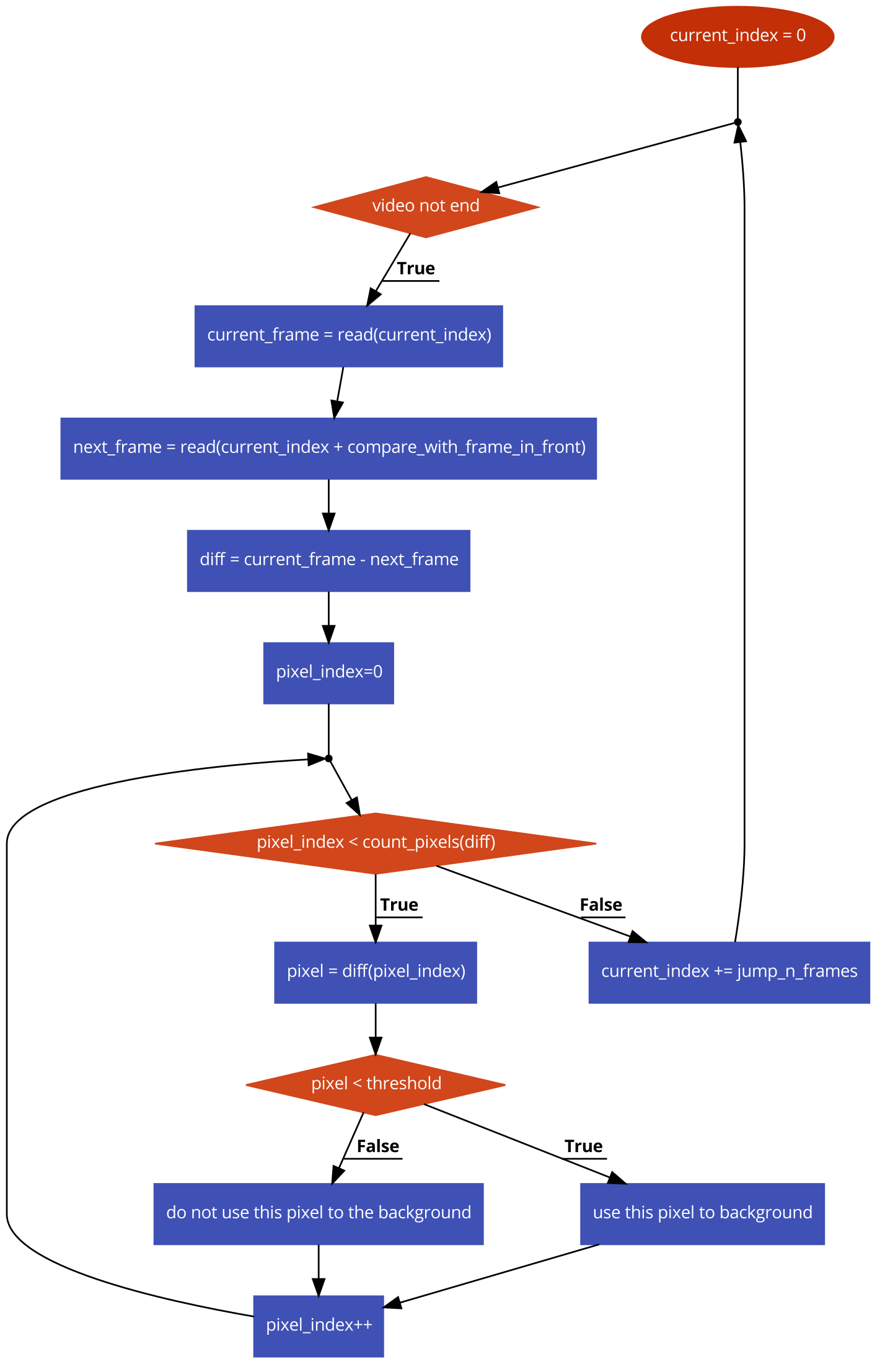# Calculate the video background¶

This module calculates the background image of the video.

## How to use¶

1. Open the “Modules” tab and choose the “Calculate the video background” module.

2. On the left check the corresponding checkboxes to select the video(s) you want to calculate the background for.

3. On the right use the sliders to change the parameters of the background calculation:

- Gaussian blur matrix size -> changes the size of the blur matrix
- Gaussian blur sigma X -> changes the sigma X of the blur matrix
- Jump n frames -> only does the algorithm described below every n frames
- Compare with frame in front -> compares the current frame with the specified frame
- Threshold -> uses the pixels over the threshold for the image

See the workflow of the algorithm to better understand what the parameters mean.

Note

Lower values for “Jump n frames”, “Compare with frame in front” and “Threshold” will result in more accurate results

4. Press the “Apply” button to calculate the background image.

## Result¶

The calculated background image will be added to the video as a new image called “background-0”.

## Algorithm¶

The algorithm used to calculate the background is described in the next workflow:## Example¶